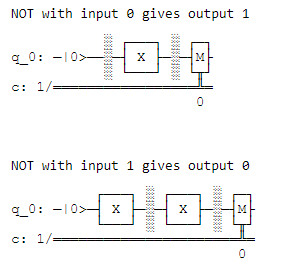# Classical NOT logic gates with quantum circuit using Qiskit in Python

• Difficulty Level : Easy
• Last Updated : 14 Sep, 2021

In this article, we are going to see how to apply NOT gate on a given input ( 0 or 1 ) using quantum gates, we have to convert 0 to 1 and 1 to 0. This can be done easily in classical computers, but how can we do this in quantum computers. We have to represent the input in qubits and then we apply the X (representation of NOT gate in the quantum computer) operation in that qubit after that return the resultant qubit

QISKIT is the package that sits between quantum algorithms from one side, and the physical quantum device from the other side. It translates the common programming languages like Python into quantum machine language. This means anyone outside of the IBM Q lab can program a quantum computer.

Attention geek! Strengthen your foundations with the Python Programming Foundation Course and learn the basics.

To begin with, your interview preparations Enhance your Data Structures concepts with the Python DS Course. And to begin with your Machine Learning Journey, join the Machine Learning - Basic Level Course

To install this module run these commands into terminal:

`pip3 install qiskit`

## Approach:

• Create a quantum circuit qc with a single classical qubit and bit using the build-in function QuantumCircuit which takes the first parameter “no. of qubits in integer” and second parameter “no. of bits in integer” and return the quantum circuit. ( QuantumCircuit(1, 1) returns quantum circuit with one qubit q and one-bit c )
• Represent input in qubits ( ‘0’ as the qubit state |0> and for ‘1’ as |1> ):
• Reset qc qubit using qiskit function reset().
• Since the qubit is initially |0⟩, so for the input of ‘0’, we don’t need to do anything.
• For an input of ‘1’, we do an X to rotate the |0⟩ to |1⟩. The x() function is to apply NOT gate on a given parameter.
• Put a barrier between input state and gate operation using barrier().
• Apply NOT on qubit 0, we can do a NOT on it using x
• put a barrier between gate operation and measurement
• Finally, we extract the |0⟩/|1⟩ output of the qubit q and encode it in the bit c using qiskit measure() function.
• To visualize and see the circuit diagram use qiskit draw() function.

Below is the implementation:

## Python3

 `# importing qiskit``from` `qiskit ``import` `*` ` ` `# importing plot_histogram to visualize Output``from` `qiskit.visualization ``import` `plot_histogram ``import` `numpy as np`` ` ` ` `def` `NOT(inp):`` ` `    ``# Creating a quantum circuit with a ``    ``# single qubit and a single classical bit using ``    ``qc ``=` `QuantumCircuit(``1``, ``1``)``    ``qc.reset(``0``)`` ` `    ``# We encode '0' as the qubit state |0⟩, and '1' as |1⟩``    ``# Since the qubit is initially |0⟩, so for``    ``# an input of 0, we don't need to do anything.``    ``# For an input of '1', we do an x to rotate the |0⟩ to |1⟩``    ``# The x() function is to apply NOT gate on given parameter.``    ``if` `inp ``=``=` `'1'``:``         ` `        ``# applying NOT on qubit 0.``        ``qc.x(``0``) `` ` `    ``# barrier between input state and gate operation``    ``qc.barrier()`` ` `    ``# Now we've encoded the input,``    ``# we can do a NOT on it using x``     ` `    ``# NOT on |0> converted to |1> and wise verse.``    ``qc.x(``0``) `` ` `    ``# barrier between gate operation and measurement``    ``qc.barrier()`` ` `    ``# Finally, we extract the |0⟩/|1⟩ output of``    ``# the qubit q and encode it in the bit c``    ``qc.measure(``0``, ``0``)``     ` `    ``# to visualize``    ``qc.draw(``'mpl'``) `` ` `    ``# To run the program on a simulator``    ``backend ``=` `Aer.get_backend(``'qasm_simulator'``)``     ` `    ``# Since the output will be deterministic,``    ``# so we can use just a single shot to get it``    ``job ``=` `execute(qc, backend, shots``=``1``, memory``=``True``)``    ``output ``=` `job.result().get_memory()[``0``]`` ` `    ``return` `qc, output`` ` `# Sending input to NOT function``for` `inp ``in` `[``'0'``, ``'1'``]:``    ``qc, out ``=` `NOT(inp)``     ` `    ``print``(``'NOT with input'``, inp, ``'gives output'``, out)``    ``display(qc.draw())``    ``print``(``'\n'``)`

Output:My Personal Notes arrow_drop_up